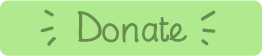# Vertical / Column Subtraction

### A demonstration tool for vertical subtraction

Learning Objectives:
• Year 2: solve problems with subtraction using concrete objects and pictorial representations, including those involving numbers, quantities and measures
• Year 2: solve problems with subtraction by applying their increasing knowledge of mental and written methods
• Year 2: to [add and] subtract numbers using concrete objects, pictorial representations, and mentally, including
• a two-digit number and ones
• a two-digit number and tens
• two two-digit numbers
This resource is designed for demonstration of formal written methods for 2-digit vertical subtraction.

The calculation is shown in a horizontal format first as children frequently find it tricky to transcribe one to the other.

The tricky columns tick box allows the random digits 1 to 9. This means that there are sometimes no tricky columns!

If you're working from home why not allow the children to set their own questions using this resource on a tablet.

Have fun!Made using theandlibraries.